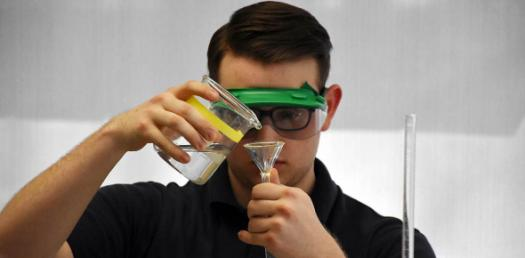# Advanced Chemistry Trivia Test! Quiz

15 Questions | Total Attempts: 39Settings.

• 1.
What is the relationship between wavelength, frequency and energy?
• A.

All direct relationships

• B.

All indirect relationships

• C.

Wavelength and energy are direct, frequency and energy are indirect, frequency and wavelength are indirect.

• D.

Wavelength and energy are indirect, frequency and energy are direct, frequency and wavelength are indirect.

• 2.
What is the shape of CO2?
• A.

Linear

• B.

Bent

• C.

Trigonal planar

• D.

Trigonal pyramidal

• 3.
What is the shape of SeCl31-?
• A.

Trigonal planar

• B.

Trigonal bipyramidal

• C.

T-shape

• D.

See saw

• E.

None of the above.

• 4.
What is hybridization?
• A.

The formation of pi bonds with p orbitals

• B.

The formation of equivalent bonds by blending different types of orbitals

• C.

The formation of hydrogen bonds between different molecules.

• D.

The formation of pi bonds with molecular orbitals

• 5.
What is resonance?
• A.

Having more than one correct lewis structure

• B.

Having more than one double bond

• C.

Having hybridization in sigma bonds

• D.

All of these

• E.

None of these

• 6.
What is the bond angle in an sp2 hybridized molecule?
• A.

90

• B.

120

• C.

180

• D.

90/120

• 7.
Which of the following are planar?
1. FClO
2. F3ClO2
3. F2ClO2
4. KrCl4
5. SeCl6
• A.

1, 2, 3 and 5

• B.

1 and 3

• C.

1 and 4

• D.

All of them

• E.

None of them

• 8.
In what ratios do atoms/ions from groups 1 and 6 combine?
• A.

1:1

• B.

1:2

• C.

2:1

• D.

2:2

• E.

None of these

• 9.
What order, from largest to smallest, would the following be ranked according to size. F, Cl-, Al3+, Na
• A.

Al3+, F, Na, Cl-

• B.

Cl-, Na, F, Al3+

• C.

Cl-, F, Na, Al3+

• D.

Al3+, Na, F, Cl-

• 10.
Which of the following would be the most electronegative metal?
• A.

Na

• B.

F

• C.

Al

• D.

H

• 11.
Which of the following would take the most amount of energy?
• A.

Removing the 1st electron from sodium

• B.

Removing the 2nd electron from sodium

• C.

Removing the 1st electron from calcium

• D.

Removing the 2nd electron from calcium

• 12.
Why does Xenon occasionally bond when most of the lighter noble gases will not?
• A.

Xenon is heavier

• B.

Xenon has more energy levels, but bonds with its inner electrons

• C.

Xenon has more electronegativity than Radon

• D.

Xenon has more energy levels and has less hold on its valence electrons

• 13.
Which requires more energy and why:  heat of fusion or heat of vaporization
• A.

Heat of fusion. Bonds are broken to form a gas and not broken when forming a liquid

• B.

Heat of fusion. Intermolecular forces are broken to form a gas and not broken when forming a liquid

• C.

Heat of vaporization. Bonds are broken to form a gas and not broken when forming a liquid

• D.

Heat of vaporization. Intermolecular forces are broken to form a gas and not broken when forming a liquid

• 14.
What is the molecular orbital notation of CO?
• A.

O1s2 o*1s2 o2s2 o*2s2 p2p4 o2p2

• B.

O1s2 o*1s2 o2s2 o*2s2 o2p2 p2p4

• C.

O1s2 o*1s2 o2s2 o*2s2 p2p2 o2p2 p*2p2

• D.

O1s2 o*1s2 o2s2 o*2s2 o2p4 p2p2

• 15.
Which of the following has a dipole moment:
1. FClO
2. F3ClO2
3. F2ClO2
4. KrCl4
5. SeCl6
• A.

1, 2, 3 and 5

• B.

1 and 3

• C.

4 and 5

• D.

All of them

• E.

None of them

Related TopicsBack to top